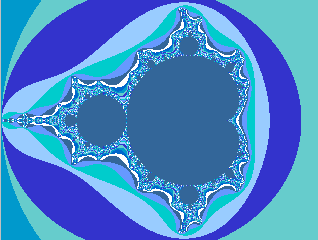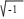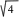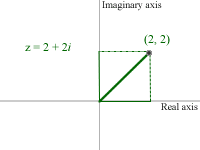Jose SandovalResume     Book     Software     Drawings     Home Search Web Search josesandoval.comFractals Page 1, Page 2, Page 3, Interactive AppletFractals are self-similar objects that sometimes are all around us: the branches of a tree, the coast line of any continent. Fractals became famous in 1985 when the first rendering of the Maldelbrot set was published in the cover of Scientific American.

What is the Maldelbrot set? The Mandelbrot set is actually a representation of the real and imaginary parts of an equation of complex numbers.

Complex numbers were invented by Mathematicians because they couldn't computelike they could. So they invented the solution for the root of -1 to be i (imaginary numbers). A complex number is represented like this z = a + bi, where a and b are Real numbers. Because our sample complex number z has two parts--a real element and an imaginary element--we can now view z as a vector in 2D. For example, if we wanted to plot z = 2 + 2i, we can do so as follows:Why is this vector representation important for fractals? Follow the next page ».Guestbook © Jose Sandoval 2004-2009 jose@josesandoval.com